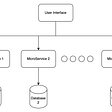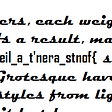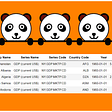# Pandas: How to change value based on condition

The values in a DataFrame column can be changed based on a conditional expression. In this tutorial, we will go through several ways in which you create Pandas conditional columns.

`import pandas as pddata = {'Stock': ['AAPL', 'IBM', 'MSFT', 'WMT'],       'Price': [144.8, 141.61, 304.21, 139.5],       'PE': [25, 21, 39, 16],         'TradingExchange': ['NASDAQ', 'NYSE', 'NASDAQ', 'NYSE']}df = pd.DataFrame(data)print(df)`

# Method1: Using Pandas loc to Create Conditional Column

Pandas’ loc can create a boolean mask, based on condition. It can either just be selecting rows and columns, or it can be used to filter dataframes.

## Syntax

`example_df.loc[example_df["column_name1"] condition, "column_name2"] = value`
• column_name1 is the column to evaluate;
• column_name2 is the column to create or change, it could be the same as column_name1
• condition is the conditional expression to apply
• value is the new value to assign

Let’s try this out by assigning the string “Under 150” to any stock with an price less than \$140, and “Over 150” to any stock with an price greater than \$150.

`df["Prcie_Category"] = "Over 150"df.loc[df["Price"] < 150, "Price_Category"] = "Under 150"`

Here is what we did:

1. We create a new column “Price_Category ” , and assign “Over 150” to every record in the dataframe.
2. Then, we use .loc to create a boolean mask on the “Price” column to filter rows where the price is less than 150. When “Price” column values meet the condition, the “Price_Category ” is assigned the new value “Under 150”.

# Method 2: Using Numpy.where

## syntax

`import numpy as npexample_df["column_name1"] = np.where(condition, new_value, “column_name2”) `

We still create “Price_Category” column, and assign value “Under 150” or “Over 150”.

`df["Prcie_Category"] = "Over 150"df['Price_Category'] = np.where(df["Price"] < 150, "Under 150", df['Price_Category'])`

We will get the same result.

But what if we have multiple conditions? we could still use .loc multiple times, but it will be difficult to understand and unpleasant to write.

# Method 3: Using Numpy.Select to Set Values Using Multiple Conditions

Now, we want to apply a number of different PE ( price earning ratio)groups:

• < 20
• 20–30
• > 30

In order to accomplish this, we can create a list of conditions

`PE_Conditions = [    (df['PE'] < 20),    (df['PE'] >= 20) & (df['PE'] < 30),    (df['PE'] >= 30)]PE_Categories = ['Less than 20', '20-30', '30+']df['PE_Category'] = np.select(PE_Conditions, PE_Categories)print(df)`

We will get the following result:

# Conclusion

In this article, we have learned three ways that you can create a Pandas conditional column. To learn more about Pandas operations, you can also check the offical documentation.

np.where

np.select

pd.loc

--

--

--

## More from Analytics Vidhya

Analytics Vidhya is a community of Analytics and Data Science professionals. We are building the next-gen data science ecosystem https://www.analyticsvidhya.com

## 8 Most Popular Architectural Design Patterns for Micro Services that any application architect must…## hackers hour CTF: write-up## How to write a very fast regex in C #. Best Practice## 2 Indices solving Minimum Distances## AWS Fargate a Lambda Function on Steroids## 12 Tips to Make Your Charts More Aesthetic## IIIF and the Problem with CSS Image Cropping.## How To Find Outliers in Data Using Python## How to Rename Column Headings with Pandas## Getting Started With Pandas## Why and How to Reshape a Pandas Dataframe from Wide to Long